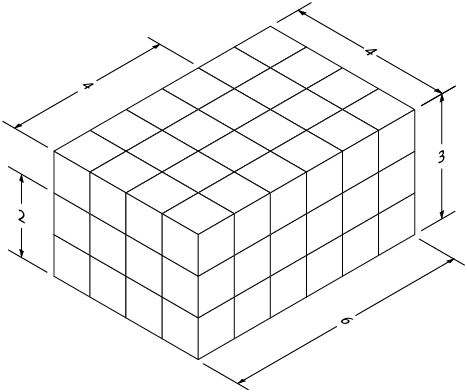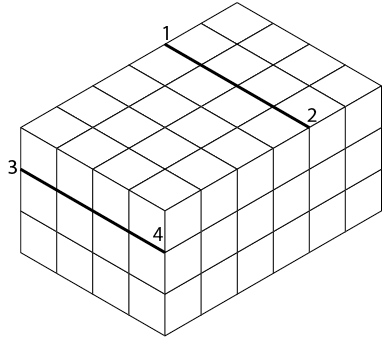# 6.3: Sketching Figures with Non-isometric Lines

Sketching figures with non-isometric lines

Figure 21 shows an object that is basically rectangular but has one face machined at an angle. You can easily construct an isometric sketch of the basic rectangular block. To show the machined face, it is necessary to plot the appropriate points of intersection and join those points to produce the correct angle.1. Rectangle with face machined at an angle

Sketch a light outline of the basic rectangular block, using the size measurements given in Figure 21. Mark the number of units indicated along the length (L) and the depth (D), as shown in Figure 22.

Lightly sketch lines parallel to the original block outlines from the marked points on the front and side surfaces, as shown in Figure 23.2. Rectangle with units marked along L and D3. Lines sketched parallel to original block outline

Join the two points on the front face and the two ends of the lines you have just sketched across the object (Figure 24). Once you are sure your sketch is correct, erase the light lines that originally outlined the block and darken the outline of the completed block as shown in Figure 25.4. Front face5. Completed block